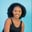Related Tags

numpy
python3

# How to find the reciprocal of a 2D array in PythonMaria Elijah

## Overview

The python library NumPy has a method called reciprocal(), which can be used to find the reciprocal of array elements.

### The numpy.reciprocal() method

The numpy.reciprocal() method returns the reciprocal of elements in a given input array.

Note: A two-dimensional (2D) array can be created using a list of lists in Python.

### Syntax

numpy.reciprocal(x, /, out=None, *, where=True)


### Parameters

• x: This represents the input array.
• out (optional): This represents the location in which the result is stored.
• where (optional): This is the condition that the input is broadcasted across. At a given location when this condition is True, the resultant array will be set to the ufunc result. Otherwise, the value of the resulting array will remain unchanged.
• **kwargs (optional): This represents other keyword arguments.

Note: If the out parameter is specified, it returns an array reference to out.

### Return value

The method numpy.reciprocal() returns the element by element reciprocal of the given input array.

### Example

The following code shows how to find the reciprocal of elements of a two-dimensional (2D) array using the numpy.reciprocal() method.

# import numpy
import numpy as np
# create a list
my_list1 =[0.5, 1, 2]
my_list2 = [5,0.7,2]
# convert to a numpy 2D array
x = np.array([my_list1, my_list2])
# compute the reciprocal of 2D array
# and store the result in array_rec
array_rec = np.reciprocal(x)

print(array_rec)
Implementing the numpy.reciprocal function

### Explanation

• Line 2: We import the numpy library.
• Line 4–5: We create two lists, my_list1 and my_list2.
• Line 7: We convert the lists to a NumPy array which gives us a 2D array. Then, the value is stored in a variable called x.
• Line 10: We use the np.reciprocal() to compute the reciprocal of elements in the x.
• Line 11: We display the result.

RELATED TAGS

numpy
python3

CONTRIBUTORMaria Elijah
RELATED COURSES

View all Courses

Keep Exploring

Learn in-demand tech skills in half the time